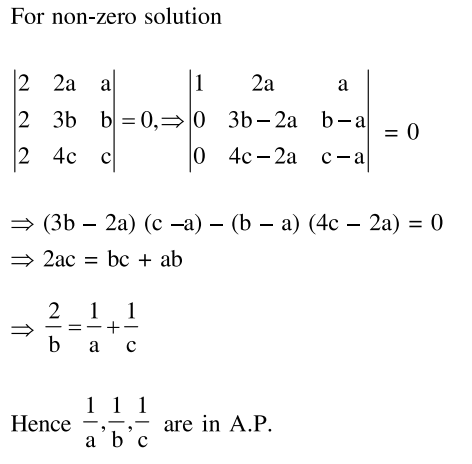# If the system of linear equationsQuestion:

If the system of linear equations

$2 x+2 a y+a z=0$

$2 x+3 b y+b z=0$

$2 x+4 c y+c z=0$

where $a, b, c \in R$ are non-zero and distinct; has a non-zero solution, then :

1. $a, b, c$ are in A.P.

2. $a+b+c=0$

3. a, b, c are in G.P.

4. $\frac{1}{\mathrm{a}}, \frac{1}{\mathrm{~b}}, \frac{1}{\mathrm{c}}$ are in A.P.

Correct Option: , 4

Solution: